Equity Valuation: Concepts and Basic Tools | IFT World
IFT Notes for Level I CFA® Program
IFT Notes for Level I CFA® Program

# Part 3

## 9. Multiplier Models and Relationship Among Price Multiples, Present Value Models, and Fundamentals

Price multiple is a ratio that uses a company’s share price with some monetary flow/value for evaluating the relative worth of a company’s stock. Commonly used price multiple ratios are listed below:

 Price multiples Ratio What it measures Price-to-earnings ratio (P/E) Trailing P/E: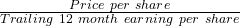For example, price = 50, EPS = 5; P/E = 10 Forward/leading/estimated P/E: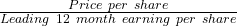Most commonly used ratio. Analysts prefer stocks with low P/E to high P/E. Price-to-book ratio P/E P/B =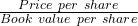Book value per share =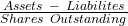Evidence suggests that companies with low P/B tend to outperform stocks with high P/B (expensive stock). Price-to-sales ratio P/S =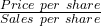Like P/E ratio, this can be trailing or leading ratio. One advantage of P/S ratio is that it can never be negative unlike P/E as earnings can be negative. It is useful during periods of economic slowdown or extraordinary growth. Price-to-cash flow ratio P/CF =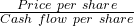One aspect to note here is what cash flow measure has been used by the analyst. The cash flow measure may be operating cash flow, free cash flow etc. Common criticism: These ratios do not consider the future. When forecast of fundamental values are used such as estimated EPS in leading P/E, the P/E value may differ substantially from the trailing P/E. When comparing companies, the multiples should be consistently used. For example, you cannot compare the trailing P/E of one company with a leading P/E of another company.

Instructor’s Note:

For a growing company, what will be higher: the leading P/E or the trailing P/E?

The trailing P/E will be higher as the earnings are higher in the future periods. So the leading P/E will be lower.

### 9.1 Relationships among Price Multiples, Present Value Models, and Fundamentals

We can link price multiple to fundamentals through a discounted cash flow model such as Gordon Growth Model. How? By assuming that intrinsic value of a security is equal to its market price i.e. the security is fairly valued.

V0 =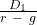becomes Pif we assume that: V0 = P0

Forward P/E =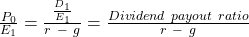The multiple you see above is related to the fundamentals as both dividend payout ratio and growth rate represent the fundamentals of a company. Some interpretations based on the formula:

• The forward P/E and payout ratio appear to be positively related. But, it does not necessarily mean a higher dividend payout increases the P/E.
• A higher payout ratio may mean the company is retaining less for reinvestment, which in turn means, a slower growth rate. Since P/E and growth rate are positively related, if g slows (denominator increases), then P/E decreases. This is known as dividend displacement of earnings.
• P/E is inversely related to the required rate of return.

Example

Between 2008 and 2012, a company’s dividend payout ratio has been 40% on average. In 2008, the dividend was $1.00 and has grown steadily to$1.8 for 2012. This growth rate is expected to continue in the future. Using a discount rate of 20%, estimate the company’s justified forward P/E.

Solution: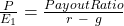The growth rate is not given. So calculate g with the information given about dividends. The growth rate is expected to continue; so it will be the long-term constant growth rate.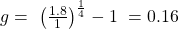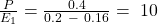## 10. Method of Comparables and Valuation Based on Price Multiples

This method compares relative values estimated using multiples. The objective is to determine if a stock or asset is fairly valued, undervalued or overvalued relative to the benchmark value of the multiple. For example, if the average P/B value for private sector banks is 1.1, and the P/B for the bank under consideration is 0.65, then it is relatively undervalued, all else equal. This method is based on the principle that similar assets should be priced the same: the law of one price.

For example, assume that there are two companies the data for which is given below:

 Company A Company B P 100 50 E1 10 6 P/E 10 8.3

On a relative value basis, company B is a better buy.

Primary difference between P/E multiples based on comparables and P/E multiples based on fundamentals:

• P/E multiple based on comparables use the law of one price. For example, if the trailing P/E of Caterpillar is 13.2, Komatsu is 15.5 and Deere is 9.6. Which one of these is undervalued? Given this data, Deere is undervalued relative to the other stocks.
• P/E multiple based on fundamentals is calculated as payout ratio/(r – g). With this method we only need information about a target company.

### 10.1 Illustration of a Valuation Based on Price Multiples

In this section, we will see through examples how price multiples is used in cross-sectional analysis, time-series analysis and in valuing a private company.

Example

The table below presents the current P/E ratio of a few automobile companies.

 Company P/E Volkswagen 12.01 Ferrari 24.57 Lamborghini 13.42 Pagani 14.1

Solution:

This is a cross-sectional analysis as different companies are compared at a specific point in time. According to the data, Volkswagen is the most undervalued as it has the lowest P/E. For every $of earnings, we are paying$12.01. It must be noted that several other factors in conjunction with relative value analysis must be performed before making a buy decision. Share prices plunge if a company is on the verge of bankruptcy.

Example

The table below computes the P/E ratio for Nikon over a five period 2012 – 2016. Determine if the stock is overvalued or undervalued relative to historic levels?

 Year Price (in \$) EPS P/E = Price/EPS 2012 17.52 1.71 10.25 2013 29.19 1.42 20.56 2014 35.7 1.2 29.75 2015 7.55 0.61 12.38 2016 5.42 0.48 11.3

Solution:

This is a time series analysis. The 2012 P/E level for Nikon indicates it is undervalued relative to the historic high of 29.75 in 2014. Analysts may recommend buying the stock if it were to return to the historic high levels provided the increase in P/E is not due to a decrease in EPS, which is not the case here. Other fundamental factors should also be considered such as is slowing revenues, the growing popularity of alternative cameras and smartphones affecting Nikon’s business, slowing economy etc.

## 11. Enterprise Value

Enterprise value is used as an alternate measure for equity; it measures the market value of the whole company (debt and equity).

Enterprise value = market value of debt + market value of equity + market value of preferred stock – cash and investments

EV = MVE + MVD + MVP – cash and cash equivalents

Note: use estimates if market values are not available

The most commonly used EV multiple is EV/EBITDA. EBITDA is earnings before interest, taxes, depreciation and amortization. It is a proxy for cash flow, or how much cash the company is generating. However, it may include other non-cash expenses and revenues.

When is EV/EBITDA used?

• When earnings are negative making P/E useless. EBITDA is usually positive.
• For comparing companies with significant differences in capital structure.
• To evaluate the cost of a takeover.

A major limitation of the enterprise value model is that it is difficult to obtain the market value of debt.

Example

The EV/EBITDA ratio for a company is 10. EBITDA is 20 million. Market value of debt is 50 million. Cash is 2 million. What is the value of equity?

Solution: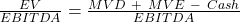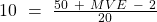MVE = 152 million

Zoom Classes for Nov-23 Exam Starting Now!
This is default text for notification bar